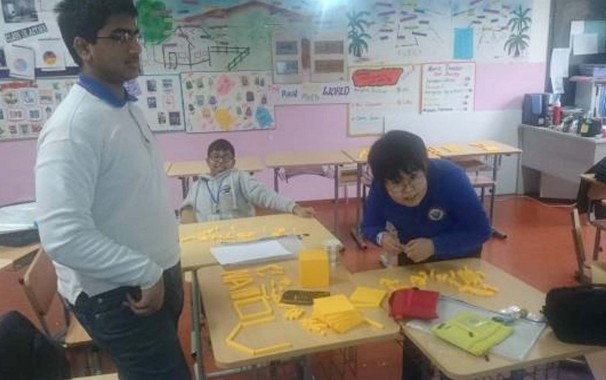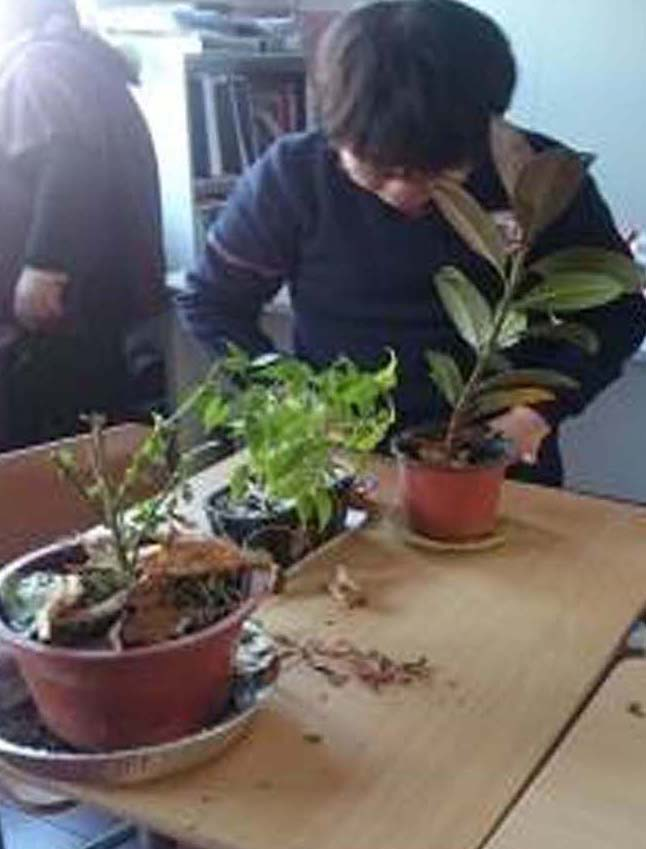08 Mar 2016
Tuesday, March 8, 2016

## Math Pre-Ap Algebra 2

NewsThis week we will cover

• Use exponential decay function and natural e to model real life situations
• Use the number e as the base of the exponential function. Write and use inverse variation
• Evaluate logarithmic functions
• Graph logarithmic function
• To model logarithmic function in real –life situations.

Goals:

• Recognize situations in which a quantity grows or decays by a constant percent rate per unit interval relative to another.
• Distinguish between situations that can be modeled with linear functions and with exponential functions
• Distinguish between situations that can be modeled with linear functions and with exponential functions.
• For exponential models, express as a logarithm the solution to abct = d where a, c, and d are numbers and the base b is 2, 10, or e;
• Evaluate the logarithm using technology.
• Interpret the parameters in a linear or exponential function in terms of a context.

Algebra 1
We will cover

• Use the discriminant to find the number of a quadratic equation

Goals:

• Choose and produce an equivalent form of an expression to reveal and explain properties of the quantity represented by the expression.
• Factor a quadratic expression to reveal the zeros of the function it defines.
• Complete the square in a quadratic expression to reveal the maximum or minimum value of the function it defines.

Pre- Algebra

SWBAT

• Analyze and solve linear equations and pairs of simultaneous linear equations
• Know that there are numbers that are not rational, and approximate them by rational numbers.
• Work with radicals and integer exponents.

Goals

• Understand that solutions to a system of two linear equations in two variables correspond to points of intersection of their graphs, because points of intersection satisfy both equations simultaneously.
• Solve systems of two linear equations in two variables algebraically, and estimate solutions by graphing the equations.
• Solve simple cases by inspection. For example, 3x + 2y = 5 and 3x + 2y = 6 have no solution because 3x + 2y cannot simultaneously be 5 and 6.
• Solve real-world and mathematical problems leading to two linear equations in two variables. For example, given coordinates for two pairs of points, determine whether the line through the first pair of points intersects the line through the second pair..
• Use rational approximations of irrational numbers to compare the size of irrational numbers, locate them approximately on a number line diagram, and estimate the value of expressions (e.g., π2). For example, by truncating the decimal expansion of 2, show that 2 is between 1 and 2, then between 1.4 and 1.5, and explain how to continue on to get better approximations.
• Use square root and cube root symbols to represent solutions to equations of the form x2 = p and x3 = p, where p is a positive rational number.
• Evaluate square roots of small perfect squares and cube roots of small perfect cubes. Know that √2 is irrational.

Math 6

SWBAT

• Use equations to solve percent problems
• Finding percent of a number
• Use percents to interpret and make circle graphs
• Find the percent of change in a quantity so you can calculate the increased sales.
• Calculate for Tax, Mark up, discount

Goals

• Use percents to interpret and make circle graphs
• Finding percent of a number
 Math Life SkillsSWBAT Calculate seed mixtures and analyze, interpret, and complete tables related to seed mixtures. Determine the costs for different seed mixtures. Analyze, interpret, and complete tables related to grass seed mixtures Goals calculate areas to determine the amount of sod needed to complete landscaping projects involving rectangles, triangles, circles, and other area configurations.